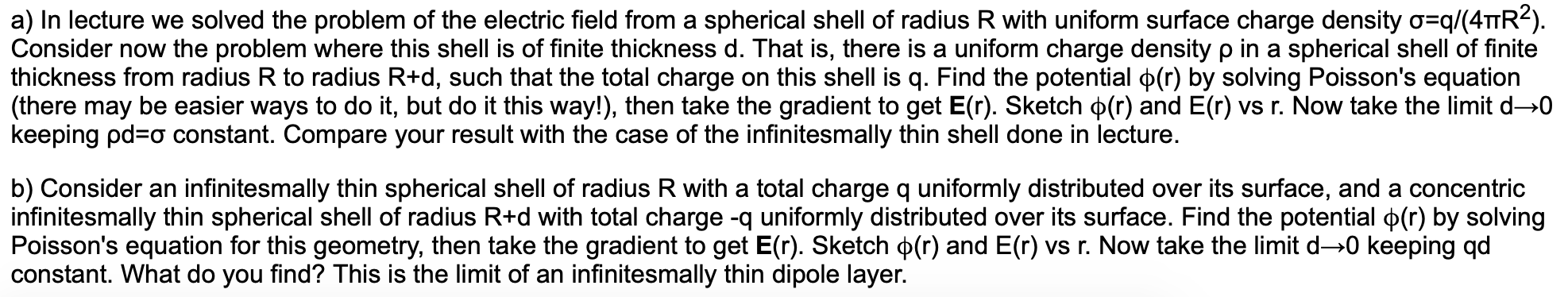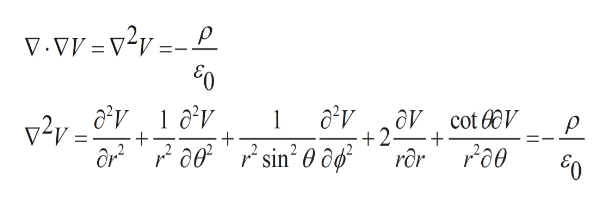# a) In lecture we solved the problem of the electric field from a spherical shell of radius R with uniform surface charge density o q/(4TTR2).Consider now the problem where this shell is of finite thickness d. That is, there is a uniform charge density p in a spherical shell of finitethickness from radius R to radius R+d, such that the total charge on this shell is q. Find the potential p(r) by solving Poisson's equation(there may be easier ways to do it, but do it this way!), then take the gradient to get E(r). Sketch p(r) and E(r) vs r. Now take the limit d-0keeping pd a constant. Compare your result with the case of the infinitesmally thin shell done in lecture.b) Consider an infinitesmally thin spherical shell of radius R with a total charge q uniformly distributed over its surface, and a concentricinfinitesmally thin spherical shell of radius R+d with total charge -q uniformly distributed over its surface. Find the potential p(r) by solvingPoisson's equation for this geometry, then take the gradient to get E(r). Sketch p(r) and E(r) vs r. Now take the limit d-0 keeping qdconstant. What do you find? This is the limit of an infinitesmally thin dipole layer.

Question
95 views

4help_outlineImage Transcriptionclosea) In lecture we solved the problem of the electric field from a spherical shell of radius R with uniform surface charge density o q/(4TTR2). Consider now the problem where this shell is of finite thickness d. That is, there is a uniform charge density p in a spherical shell of finite thickness from radius R to radius R+d, such that the total charge on this shell is q. Find the potential p(r) by solving Poisson's equation (there may be easier ways to do it, but do it this way!), then take the gradient to get E(r). Sketch p(r) and E(r) vs r. Now take the limit d-0 keeping pd a constant. Compare your result with the case of the infinitesmally thin shell done in lecture. b) Consider an infinitesmally thin spherical shell of radius R with a total charge q uniformly distributed over its surface, and a concentric infinitesmally thin spherical shell of radius R+d with total charge -q uniformly distributed over its surface. Find the potential p(r) by solving Poisson's equation for this geometry, then take the gradient to get E(r). Sketch p(r) and E(r) vs r. Now take the limit d-0 keeping qd constant. What do you find? This is the limit of an infinitesmally thin dipole layer. fullscreen
check_circle

Step 1

The spherical shell having radius R and thickness t is shown below. The potential is related to the charge density by Poisson’s equation as shown.

Step 2

The spherical shell having thickness t, its polar spherical  coordinates is given by  Poisson’s equation:help_outlineImage Transcriptionclose= v2y_=_P 50 iv v v aV cot V 2 ror 1 00sin2 0 ôd* or fullscreen
Step 3

Due to symmetry in the hollow spherical shell the derivative of terms cont...

### Want to see the full answer?

See Solution

#### Want to see this answer and more?

Solutions are written by subject experts who are available 24/7. Questions are typically answered within 1 hour.*

See Solution
*Response times may vary by subject and question.
Tagged in

### Electrical Engineering